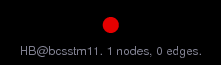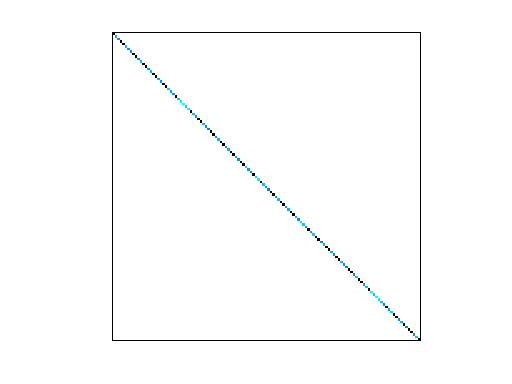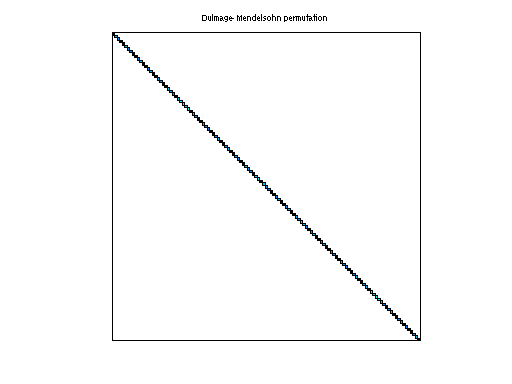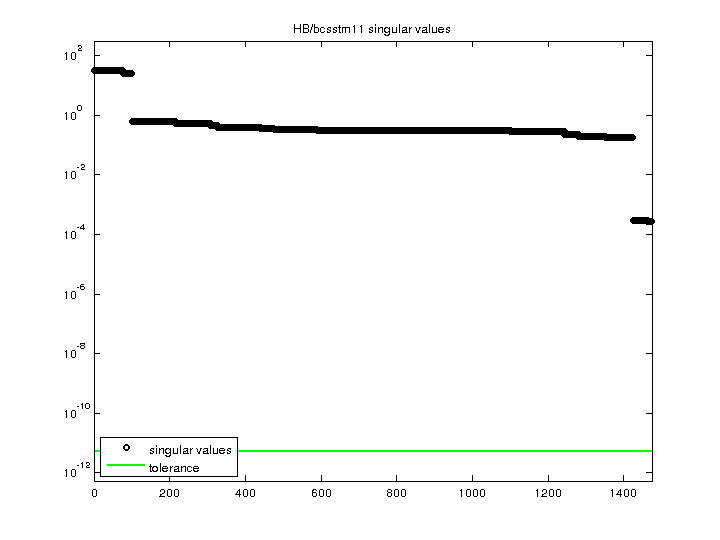Matrix: HB/bcsstm11

Description: SYMMETRIC MASS MATRIX, ORE CAR (LUMPED MASSES)(undirected graph drawing)• Matrix group: HB
• download as a MATLAB mat-file, file size: 5 KB. Use UFget(66) or UFget('HB/bcsstm11') in MATLAB.

 Matrix properties number of rows 1,473 number of columns 1,473 nonzeros 1,473 structural full rank? yes structural rank 1,473 # of blocks from dmperm 1,473 # strongly connected comp. 1,473 explicit zero entries 0 nonzero pattern symmetry symmetric numeric value symmetry symmetric type real structure symmetric Cholesky candidate? yes positive definite? yes

 author J. Lewis editor I. Duff, R. Grimes, J. Lewis date 1982 kind structural problem 2D/3D problem? yes

 Ordering statistics: result nnz(chol(P*(A+A'+s*I)*P')) with AMD 1,473 Cholesky flop count 1.5e+03 nnz(L+U), no partial pivoting, with AMD 1,473 nnz(V) for QR, upper bound nnz(L) for LU, with COLAMD 1,473 nnz(R) for QR, upper bound nnz(U) for LU, with COLAMD 1,473

 SVD-based statistics: norm(A) 31.5781 min(svd(A)) 0.00026705 cond(A) 118248 rank(A) 1,473 sprank(A)-rank(A) 0 null space dimension 0 full numerical rank? yes

 singular values (MAT file): click here SVD method used: s = svd (full (A)) ; status: ok